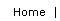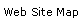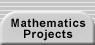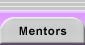# EXAMPLES, PATTERNS, AND CONJECTURES

Mathematical investigations involve a search for pattern and structure. At the start of an exploration, we may collect related examples of functions, numbers, shapes, or other mathematical objects. As our examples grow, we try to fit these individual pieces of information into a larger, coherent whole. We note common properties of our examples and wonder if they apply to all possible examples. If further testing and consideration lead us to strengthen our belief that our examples reflect a more general truth, then we state a conjecture. The Latin roots of "conjecture" translate to "throw together"—we are throwing together many observations into one idea. Conjectures are unproven claims. Once someone proves a conjecture, it is called a theorem.

You can introduce the ideas and activities discussed below as the need for them arises during student investigations. If a student uses a particular technique, highlight that approach for the class. Once a conjecture is posed, ask the class what they need to do to understand it and begin to develop an outline that all can use. Regular opportunities for practice with the different skills (organizing data, writing conjectures, etc.) will lead to greater student sophistication over time.

## GENERATING AND ORGANIZING EXAMPLES

### Generating Examples

In order to get a better view of the "big" picture of a problem, we try to produce examples in a systematic fashion. We often have to choose examples from an infinite domain. These examples should be representative, in ways that we deem significant, of all of the elements of the domain. For example, a problem involving real numbers might involve positive, negative, whole, rational, and irrational examples. Numbers that are less than one or of great magnitude might also be important. In addition to this broad sampling, we also want to generate examples in a patterned way so that relationships between variables stand out (see Organizing Data below).

For some problems, examples are easy to produce. At other times, it is not clear if the objects described even exist or, if they do exist, how to construct them. For example, a student interested in the parity of the number of factors for each counting number might have difficulty finding numbers with an odd number of factors. Her search for examples will probably lead her to wonder why most numbers have an even number of factors and perhaps guide her to the conditions that yield an odd number of factors. This intertwining of discovery and understanding is common throughout mathematical work—proofs often co-evolve with the discoveries themselves.

It is important to determine when examples are actually different from one another. If we are unable to state what characteristics really matter for a particular problem (e.g., order or shape), then we will not be able to figure out when we have enough examples, whether any others remain to be found, or what the sample space that we are searching is. For example, students may find it challenging to generate a diagram that matches the following conditions or to determine whether their examples are even distinct from each other:

Draw a map showing towns and roads such that:
• Each pair of roads has exactly one town in common.
• Each pair of towns has exactly one road in common.
• Every town is on exactly three roads.
• Every road contains exactly three towns.

As neighbors compare their maps, ask them to consider in what ways the maps differ and in what ways they match. What characteristics count when they consider two maps to be the same? As with the rectangle problem below, we often attend to the topology of a mathematical object more than to its exact measurements. An object’s topology is dependent on how its parts are connected to each other.

### Being Systematic

We may find many solutions to a problem but still miss interesting ones if we are not systematic in our search. In order to be systematic, we have to create a path or paths that will take us through all of the possibilities that might arise. Staying on the path may require an algorithm that guides us through the choices that we face along the way. The algorithm itself may not be apparent until we have tried to generate an ordered list and omitted or over-counted some examples. Only, after first experimenting, may we start to understand the internal logic of a problem.

For practice, students can consider the following question:

A class is investigating subdivisions of a rectangle into n smaller rectangles. They are working on the specific case of dissecting a rectangle into 4 rectangles. What layouts are possible for these subdivisions?

A complete search for even this small case of four rectangles requires careful reasoning. We can consider all possibilities more efficiently by picking a single corner as our starting point. Recognizing the symmetry of the situation (a rotation or reflection makes the chosen corner equivalent to the other three) simplifies our work. There are two ways to put a rectangle in this corner: along an entire side or not (figure 1). Again symmetry comes to our aid—it does not matter whether the entire side that we cover is oriented horizontally or vertically.

Of course, if we are going to appeal to symmetry, we have to define what we mean by a distinct answer. It is clear that there will be an infinite number of solutions if the size of the subdivisions is taken into consideration. So, it makes sense to ask how many categories of these subdivisions there are when we ignore the size of segments and the overall orientation of the figure and just look at the topological relationship between the sub-rectangles (how they border on one another).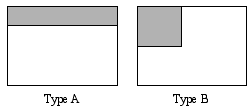Figure 1. The first rectangle is placed in the top left corner

Once we have the two starting arrangements, we have to add three more rectangles. For the rectangle on the left, we are just left with a smaller version of our original problem—dissecting a rectangle (the remaining space) into three rectangles. There are only two different ways to perform such a dissection (test this claim yourself!). We can rotate these three-rectangle arrangements to generate new candidates for subdivisions using four rectangles (figure 2). One duplicate solution arises (the crossed-out picture is a equivalent to the one in the upper right corner), so there are five variations thus far.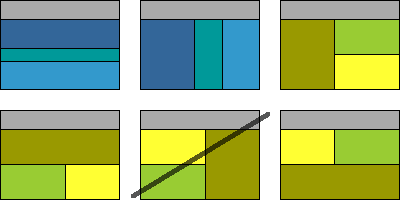Figure 2. Completing a type A rectangle

We can complete the type B rectangle in two additional unique ways (figure 3).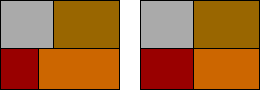Figure 3. Completing a type B rectangle

Another valuable technique for generating examples is to build them up inductively from those of a smaller case. We can produce the seven subdivisions found above by bisecting one sub-rectangle in the three-rectangle subdivisions (figure 4).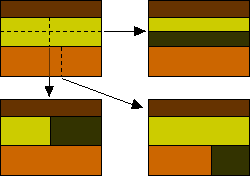Figure 4. Three rectangular subdivisions are turned into four

This inductive approach works nicely when finding all polyominoes made using n squares from the set of polyominoes made with n – 1 squares. The n-square polyominoes are found by adding one additional square to each available edge of those made with n – 1 squares. However, this inductive approach does not work dependably for the rectangle subdivision problem. Subdividing one rectangle of a four-rectangle layout cannot generate the five-rectangle subdivision pictured below (figure 5). This problem demonstrates that we must thoughtfully choose the methods that we use to generate examples if we want to identify all cases of interest.

See Testing Conjectures, below, for a further discussion of different types of examples.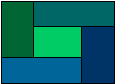Figure 5. A special subdivision

### Organizing Data

The examples that we produce in our investigations provide us with data. We try to organize that data in a way that will highlight relationships among our problem’s variables. Although there are no guaranteed methods for discovering all patterns, there are some useful basic methods. Numerical data can be organized in tables that facilitate our search for familiar patterns. In a problem with two variables, one dependent on the other, the information should be listed according to constantly increasing values of the dependent variable. For example, a student wondered about the number of regions formed by the diagonals of a regular n-gon. She systematically listed the number of sides of the polygons and the number of regions created (figure 6). This essentially one-dimensional arrangement facilitates the discovery of any recursive or explicit functions that relate the two variables.

 n Regions 3 1 4 4 5 11 6 24 7 50

Figure 6. A table of the number of regions made by the diagonals of a regular n-gon

Sometimes a problem will have several independent variables (the values that they can take are not constrained by the other variables). In such cases, we can organize our data by using each dimension of a table to represent the values of one variable. For example, in 1899, Georg Pick published a formula that gave the area for a polygon whose vertices lie on the points of a square lattice (figure 7). He discovered that the area could be determined based solely on the number of lattice points within the interior (I) and on the boundary (B) of the polygon. His formula was A = 1/2B + I – 1.Figure 7. A polygon with 2 interior and 10 boundary points has area 2 + 1/2(10)1 = 6

There are several steps one might take toward making such a discovery. One might begin with the intuitive notion that the area of a region might be related to the number of lattice points inside the figure. Then one needs the insight that the area might be a function of just two variables—that it does not depend on the particular shape of the polygon or the number of vertex points. Perhaps this conjecture arises by looking at several examples for the same B and I. Once this fact is discovered, areas can be found for shapes with different combinations of boundary and interior points (figure 8). A class can use this table to try to find Pick’s formula themselves. Have them draw, or use geoboards to make, shapes that meet the requirements of a given (B, I) pair and find the areas of those shapes. (Note: finding the area can be a good challenge all by itself—a potentially helpful suggestion is to subdivide the polygon into simpler shapes or draw the rectangle that circumscribes the shape and subtract away excess areas).

 Points in the interior (I) Boundary points (B) 3 4 5 6 … 0 1 2 3 …

Figure 8. A two-dimensional table for organizing data for Pick’s theorem

As the table is filled in (figure 9), students may note various patterns. They may state what they see recursively (e.g., "You add one half each time you move over a box.") or explicitly ("When B is four, the area is one more than the number of inside points."). Both of these forms can be helpful in developing a general solution. Encourage your students to write formulas for each row and column and then try to combine these sub-rules into one that works for all (B, I) pairs. It is important for students to realize, however, that their formulas are conjectures rather than theorems. A proof that the formula always works is a very challenging task that cannot be based on the data from a finite number of examples (but you might encourage them to try to prove it for easier special cases of polygons such as rectangles, right triangles, etc.).

 Points in the interior (I) Boundary points (B) 3 4 5 6 … B 0 1/2 1 1 1/2 2 1 1 1/2 2 2 1/2 3 B / 2 2 2 1/2 3 3 1/2 4 3 3 1/2 4 4 1/2 5 … I I + 1/2 I + 1

Figure 9. A two-dimensional table for organizing data for Pick’s theorem

When a problem has many variables, or a large number of possible values for each variable, it can be difficult studying all combinations systematically. In such cases, we can choose to hold one (or up to all but one) variable constant and study the others. In doing so, we are changing the problem to one of a more manageable size. For example, in the Pick data above (figure 9), a student might first study the column for 4 boundary points (B is held constant while I is allowed to vary). The rule that describes that column, I + 1, does not, by itself, give us a formula for the entire table. We must also hold our first variable, B, constant for some new values and then repeat our analysis for fixed values of I while varying B.

The Connect the Dots research setting provides an additional example of the value of temporarily holding a variable constant. Students typically hold the dot number constant and generate the diagrams for all jump sizes. Ultimately, we hope to fit all of our findings from these narrower investigations into one larger result that solves the original problem. For examples of graphical approaches to displaying data to find connections, see Graphs in Representations.

## LOOKING FOR PATTERNS

We organize the observations that we make in order to develop conjectures about the behaviors of the mathematical objects that we are studying. Once we believe that a pattern is established, we will state it as a conjecture about an entire class of objects.

### Variables That Change Predictably

As we organize our data, we look for patterns and for ways of describing those patterns formally. Students learn about a variety of familiar patterns (linear, exponential, periodic, etc.) in their algebra classes. Techniques for identifying these patterns and activities that help them develop the habit of using these techniques should be central to this study. A good resource for teaching the characterization of different patterns is the first chapter of Mathematical Methods (http://www.its-about-time.com/htmls/mm/mm.html) soon to be published by Its About Time (http://www.its-about-time.com).

#### Simplifying a Problem

Problems can be so intellectually challenging or computationally demanding that we cannot solve them directly. For example, a student reading Flatland wondered about the lengths of the diagonals of a hypercube. What were the longest segments that could fit in such a figure? She began by looking at the lower dimensional versions of the problem. For a point, which is a 0-dimensional "cube", the length of the longest fitting segment is 0. For a unit segment (the 1-dimensional "cube"), the length of the longest segment is 1. For a unit square, we can fit a diagonal of length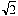and for a unit cube, the distance from one corner to the opposite one is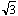(figure 10). Recognizing that 0 and 1 are both their own square roots, she extrapolated her pattern and decided that the diagonal of a unit, 4-dimensional hypercube must be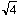or 2. Her pattern did not constitute a proof, but the study of simpler related cases guided her to a solution and, ultimately, to a proof for any dimension.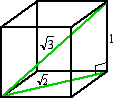Figure 10. The diagonals of a unit segment, square, and cube

In the hypercube example above, the student used smaller cases because she could not, at first, visualize the situation that interested her. Using a smaller case is especially important if you find yourself attempting a brute force solution to a problem. For example, instead of counting all of the possibilities for a five button simplex lock, one of the project hints is to first find a pattern for locks with fewer buttons.

Problems can be made simpler using a smaller number, simpler shape, or more symmetric setting or shape (e.g., a square rather than an arbitrary quadrilateral). They can sometimes be made simpler by removing restrictions that seem to make them harder. For example, the problem "In how many different ways can an elevator leave the ground floor of a 20 story building, make 10 stops moving only upward, and arrive at the top floor?" is more difficult than the problem that allows the elevator to move in either direction during its stops. For a further discussion of methods for creating related problems, see Ways to Change a Problem in Problem Posing. For a class activity that raises questions about how a simpler problem can be used to solve a more complex one, see the section Technology and Magnitude in the Numbers in Context chapter from the book Mathematical Modeling available from www.meaningfulmath.org (note: this chapter is a 1 megabyte downloadable pdf file).

### Invariants – Quantities, Objects, and Relationships That Stay the Same

While we often seek to describe how some variable is changing, sometimes we want to show that a feature is unaffected by changes in a variable. For example, the ratio of the circumference of a circle to its diameter remains unchanged even as the circle’s size varies. This fact is obvious once you study it, but can be a surprise to children when they first discover it. A property or quantity that does not change while other variables are changing is called an invariant. The notion of invariance is important throughout mathematics.

Even shapes much less constrained in their form than circles have invariant properties. For example, if we construct squares on the sides of a quadrilateral, the segments connecting the centers of the squares on opposite sides will be both equal in length and perpendicular (Aubel’s theorem, figure 11). Invariants often prove surprising and stimulate further investigation and explanation. When such consistency appears in the face of asymmetry and variability, we want to find out what accounts for this dependability.Figure 11. For any quadrilateral, the blue segments are equal and perpendicular

The identification of invariants can be a very powerful tool because it lets us find common properties of situations that look very different. The identification and use of invariants becomes more natural with experience. Here are two examples that provide a fuller picture of this concept:

An Algebraic Invariant — Consider the polynomial equation  0 = x2 + x + a  with a constant. We can use the quadratic formula to find the solutions: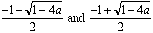. The sum of these solutions is –1. Therefore, the sum of the solutions to 0 = x2 + x + a  is invariant with respect to a. Similarly, you can show that the sum of the solutions of 0 = cx2 + bx + a  is invariant with respect to the constant term a and that the product is invariant with respect to the linear coeeficient b.

A Combinatorial Invariant — Suppose we start with a permutation (or reordering) of the numbers 1 through 9:

{6, 8, 1, 9, 3, 7, 5, 4, 2}

There are many different ways to arrange these numbers in ascending order by exchanging pairs. You could start by exchanging the 9 and 2 to give you:

{6, 8, 1, 2, 3, 7, 5, 4, 9}

Someone else might exchange the 8 and the 2 in the original ordering to get

{6, 2, 1, 9, 3, 7, 5, 4, 8}

No matter what you do first, keep switching pairs until a final exchange produces

{1, 2, 3, 4, 5, 6, 7, 8, 9}

Try this with some numbered tiles or scraps of paper and keep track of the number of exchanges that you make. The number of steps might vary, but you will always take an odd number of steps. We say that the parity of the original permutation is odd as opposed to being even if sorting the numbers took an even number of steps, as is the case for {5, 2, 4, 9, 1, 3, 6, 7, 8,}. The parity of a permutation is invariant with respect to the exchanges you perform to sort the permutation.

#### An Activity with Invariants

Consider the following problem (from Breaking Chocolate Bars at http://www.cut-the-knot.com/proofs/chocolad.html) involving a candy bar that divides into m by n small squares (figure 12). Starting with a whole bar, a move consists of choosing a piece of the bar and breaking it along one of the horizontal or vertical lines separating the squares. The two new pieces are then returned to the pile to be available for the next move. The challenge is to find the fewest number of moves needed to break the bar into all individual (1 by 1) squares.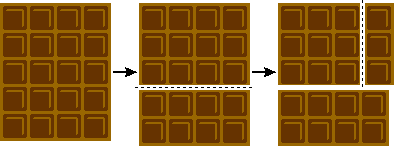Figure 12. A 4 by 5 chocolate bar and two possible first moves

Introduce this situation to your class and have each pair of students pick bars of a particular size and keep track of the number of moves needed each time. They can carry out their investigations cutting pieces of graph paper, separating sections of an array of interlocked cubes, drawing segments on a grid, or breaking an actual chocolate bar. Ask them to consider whether certain strategies for choosing which piece to break seemed more efficient than others.

After several tries, it should become apparent that, for a given size bar, the number of moves required is always the same. Why is the outcome invariant with respect to the sequence of breaks? What does affect the number of moves needed? At this juncture, you can guide your class in one of two directions. They can be asked what variables affect the number of moves and be given time to find a rule that predicts that total—they may think about organizing the possibilities for different values of m and n in an array or try looking at specific small cases. Alternatively, and this is the faster route, you can ask them to keep track of the number of pieces after each cut. You may even want to do both of these analyses in order.

The key observation is that the number of pieces of candy is always one more than the number of moves because each move adds one more piece. That is, the number of pieces is invariant with respect to the choice of breaks made. This invariance tells us that we will always end up with mn pieces after mn – 1 moves. This activity provides a nice example of how we can use invariants as a tool for constructing proofs.

For additional teaching material related to invariants, see Chapter 1 lessons 7, 8, and 10 in the book version and lessons 14, 15, and 16 in the CD-ROM version of the Connected Geometry curriculum (http://www.everydaylearning.com/geometry). You can also download Tackling Twisted Hoops, an article on invariants and knot theory from the former Quantum magazine.

### A Different Kind of Observation

Although the most common kind of discovery for secondary students engaged in mathematics research to make is one about numerical patterns, there are other kinds of possible conjectures. One type of observation could be that a pattern or arrangement that they are studying has been encountered in another context. Such an observation can lead to a conjecture that there is a common explanation for the two apparently dissimilar questions and to a way of showing that the two are related in some manner.

One such inquiry began when another student who was inspired by Flatland looked at the number of vertices in an n-dimensional cube a given distance away from a chosen vertex. The distance was measured by travelling only along the edges of the figure. For example, if a corner of a square is chosen, then there is one point 0 steps away (the point itself), two vertices that can be reached by travelling along a single edge, and one vertex a distance of two edges away (figure 13).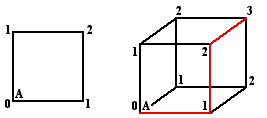Figure 13. The distance along the edges from vertex A in a square and a cube

When the student organized his data in a table (figure 14), he saw a familiar sight. He then conjectured and set out to explain the connection between n-dimensional cubes and Pascal’s triangle.

 d Point Segment Square Cube Hypercube 0 1 1 1 1 1 1 0 1 2 3 4 2 0 0 1 3 6 3 0 0 0 1 4 4 0 0 0 0 1

Figure 14. The number of vertices distance d away from a vertex in each shape

For further discussion and settings that encourage the making of such connections, see Practice Activities in the Proof section.

## UNDERSTANDING CONJECTURES

We seek to understand a conjecture at three levels: we want to determine its meaning, we want to identify reasons for why we might believe the claim to be true, and we want to understand how it fits within some larger set of ideas. The initial steps we take when exploring a conjecture are similar to those used to understand a definition:

1. Read the statement more than once. Important subtleties are often missed on the first read.
2. Identify each of the conditions of the conjecture. A conjecture’s conditions are those criteria that must be satisfied before we accept the conclusions of the conjecture. They are the if part of the statement. Each noun and adjective may constitute a specific condition.
3. Generate examples and non-examples. Find objects that meet the conditions and check to see that they also satisfy the conclusion of the conjecture. Remove each condition in turn and construct non-examples that satisfy the other conditions but not the conclusion. Non-examples help us understand the importance of each condition to the conjecture. Conditions constrain the objects under consideration to a set that all share particular properties.
4. Look for counterexamples. A counterexample satisfies all conditions of a statement but not the conclusion. Do the conditions leave "wriggle room" for an object that fails to satisfy the conclusion of the conjecture? If a counterexample does exist, then the conjecture is false.
5. Compare. How is this conjecture related to other statements about the same or similar mathematical objects?

The same steps help when we are familiarizing ourselves with a new theorem. In the case of a theorem, we want to read and understand the proof as well. In the case of a conjecture, we are looking for evidence that would support a proof or provide a path to a disproof.

Removing conditions

The Angle-Angle-Side (AAS) Congruence Theorem: If, in two triangles, two angles and a non-included side of one triangle are congruent respectively to two angles and the corresponding non-included side of the other, then the triangles are congruent.

The AAS theorem has many conditions. It involves triangles, two angles, one side, the side’s position relative to the angles, congruency, and correspondence. In order to see why each one is necessary, we need to remove each condition and then create a pair of triangles that satisfy the remaining conditions but are not congruent. For example, if we remove the condition that the figures are triangles, we can construct different quadrilaterals that share two congruent angles and an equal non-included side. The table below (figure 15) shows non-examples for three different conditions.

 Condition Removed Non-examples Figures are triangles.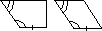Two angles must be congruent.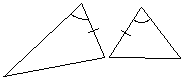Congruent side must be the corresponding non-included one (these triangles are similar but not congruent).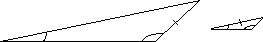Figure 15. Non-examples for the AAS theorem

As a class activity, present theorems and conjectures and ask students to first list all conditions of the statement and then produce non-examples for each. Two claims that they can practice with are (1) If a and b are positive integers, d is the greatest common factor of a and b, and c is not divisible by d, then there are no integral solutions to ax + by = c and (2) If an odd integer is raised to an odd integral power, then the result is an odd integer. Do not tell your class ahead of time that the first claim is a theorem while the second is a false conjecture. Students will get additional practice understanding the conjectures that their peers generate throughout the year.

## EVALUATING CONJECTURES

What are the possible characteristics of a conjecture and what makes one conjecture more interesting than others? Students should explicitly answer each of the following questions when they seek to evaluate a conjecture:

• Does the conjecture appear to be true or false?

We rarely have a definitive answer to this question right away, but our understandings of related results may guide our intuitions. A study of examples and a search for counterexamples will further influence our belief in the truth of a conjecture. Students tend to be too prone to believe that a few examples constitute irrefutable evidence of a pattern.

• Is it obvious or subtle?

If a conjecture immediately follows from a known result, then it may be less interesting than an unexpected conjecture. For example, a claim about squares may not be exciting if a student has already proven the same claim for a superset such as parallelograms.

• Is it easy or difficult to understand?

A conjecture may be difficult to understand because of the way it is written or because the mathematics involved is inherently complicated. Students should re-write their conjectures if their mathematical language is unnecessarily confusing.

• Is the conjecture general?

A conjecture that, if true, applies to a broad range of objects or situations will be more significant than a limited claim. Does it suggest a connection between two different topics?

• Is it specific enough?

A second-grader was investigating which n by m boards could be tiled by the T-tetromino (four squares arranged edge-to-edge to look like a capital T, figure 16).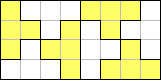Figure 16. A 4 by 8 board tiled with T-tetrominoes

Her ultimate conjecture, "If the sides of a board are even by even it may work, if not then not," left her dissatisfied. She correctly believed that any odd dimension made the tiling impossible, but she knew that evenness was not a precise enough condition to distinguish between all of the boards that did and did not tile. She knew that if she could refine her conditions her conjecture would be stronger. (See Necessary and Sufficient Conditions below.)

• Do you like the conjecture? Do you find it appealing?

People are attracted to different mathematics questions. It is important for students to begin to develop their own aesthetic for mathematical ideas and to understand that aesthetics play a role in the discipline. Conjectures that are unexpected or counter-intuitive, that reveal a complex pattern, or that would be useful in supporting other important conjectures are more likely to be appreciated by a wide audience.

## TESTING CONJECTURES

The first question that we face in evaluating a conjecture is gauging whether it is true or not. While confirming examples may help to provide insight into why a conjecture is true, we must also actively search for counterexamples. When students believe a conjecture, they are not always rigorous in their search for examples that break the pattern that they have identified. We must help them develop the habit of being more skeptical. One way we can develop this skepticism is by giving students problems that have "false" patterns—ones that seem familiar but do not continue as expected (see Conjectures Are Not Theorems below).

How can students search for counterexamples? They should test cases between those that they have found to work. They should look at extreme cases at the far ends of the domains of their problems (e.g., obtuse triangles that are nearly flat or numbers near zero). They should consider degenerate cases that do not have all of the complexity of a typical example. Degenerate cases often result from making some parameter zero. For instance, a point when the conjecture applies to circles (the radius has been set to zero) or a linear equation when the topic is quadratics (the coefficient of the squared term is zero). A quadrilateral is a degenerate pentagon in which two of the points are in the same location. Of course, some degenerate cases are not really relevant to a problem (e.g., ellipses can be defined in terms of a focus and directrix, but circles cannot).

In addition to extreme and degenerate examples, we should also generate and test special cases. Special cases possess an additional property, such as symmetry, that most other cases lack. A square is a special rectangle and right or isosceles triangles are distinct cases to investigate. In other contexts, special cases might be numbers with no duplicate prime factors, matrices with a determinant of 0 or 1, or functions that are monotonic.

Before a class investigates new conjectures, I present them with a former student’s summary: "A conjecture has three possible fates: life, death, and limbo. Life is gained through proof, death by counterexample, and limbo is just limbo. Death can lead to rebirth through refinement." This pithy statement emphasizes that a single counterexample is sufficient to kill a conjecture and that proof—not a slew of examples or the absence of a counterexample—is the only way we can be certain of a claim.

Students should not see the discovery of a counterexample as a failure. It is both a sign of their thoughtfulness and the possible start of a new and better theory. A counterexample may disprove a conjecture, but it does not mean that the claim is always false. A conjecture may be nearly always true. Conjectures are reborn when we identify what extra condition removes the possibility of counterexamples or what part of our conclusion we need to make less specific. For example, a geometry student’s conjecture that "the intersections of the angle bisectors of a parallelogram form a rectangle in the interior of the parallelogram" was salvaged by classmates who pointed out that the word "interior" made counterexamples possible. Another students’ conjecture, "The perpendicular bisectors of the sides of a kite do not intersect in a point," works for most kites but needed the additional condition that the non-end angles not be right angles. Students should group their counterexamples and confirming examples and look for a property that distinguishes the two sets.

For a class activity that involves looking for counterexamples, see the section Understanding Definitions: Closed and Dense in the Numbers in Context chapter from the book Mathematical Modeling.

## WRITING CONJECTURES

For students, there is often a wide gulf between the ideas with which they can grapple and their ability to write a clear statement of their thinking. They need to learn the mathematical vocabulary and formal structures that make writing a logical claim easier. They make that progress when we provide them with ample opportunity to recognize, write, and refine conjectures. The following describes a sequence that provides practice creating and reworking conjectures.

### Class Activity

Dynamic geometry programs, such as Geometer’s Sketchpad ® or Cabri Geometry II ™, that allow students to construct accurate diagrams of geometrical objects have become popular tools for classroom investigations. If students are given some flexibility in the settings that they explore, they will happily struggle with complicated conjectures that can be a valuable focus of class discussion. Consider the following sampling of claims, ranging from grand to muddled, that were generated by students. The conjectures were responses to an assignment to choose a class of quadrilaterals and to identify any properties of the angle bisectors or perpendicular bisectors of the sides of those figures.

• The intersections of the perpendicular bisectors of a parallelogram create a new parallelogram with the same angle measures as the original.
• The angle bisectors of a rectangle make a square.
• All of the perpendicular bisectors of an isosceles trapezoid intersect at the same point. That point of intersection is the center of a circle which contains all of the vertices of the isosceles trapezoid.
• In a trapezoid, the angles formed at the intersection point of the longest base line and the non-base angle bisector are congruent only if both angles are outside or both are outside.
• In an isosceles trapezoid, the base angle bisectors create an angle equal to the opposite base angle.
• In a trapezoid, two congruent triangles are made by the bisectors of consecutive non-base angles and where one intersects the base line.
• In a kite, where all the angle bisectors meet is the center.
• In a trapezoid, the angles formed at the intersection point of the longest base line and the non-base angle bisector are congruent only if both angles are inside or both are outside.
• In a trapezoid, the two base angle bisectors make an isosceles triangle at the point of intersection.
• If the angle bisectors of a quadrilateral form a rectangle, then that figure is a parallelogram.
• An isosceles trapezoid’s angle bisectors create four congruent right triangles.

After two or three days of laboratory time, each group submits their lab report with examples and conjectures. To make sure that they clearly define what they are describing, they are not allowed to use labels from the figures; they had to use accepted vocabulary. The next day each student is given a page of conjectures, such as the above list, drawn from the reports. They read the conjectures and try to understand them, to generate test cases, and to evaluate them according to the standards noted above (see Evaluating Conjectures).

The class then discusses each conjecture. Anonymously, students listen to what their peers think about the clarity of their writing and the success of their conjecture. Students provide constructive advice on how to clarify each statement. An advantage of peer review such as this to teacher comments is that students are usually quite good at finding alternative interpretations of confusing statements that reveal the imprecision in a claim. Often, a class will have several interpretations among which it cannot decide. This predicament helps the author figure out how to modify their conjecture to say what they intended. A cycle of peer evaluation and rewriting leads to better conjectures and better self-edited conjectures in the future.

Peer review of first drafts is preferable to teacher evaluation because teachers are too good at figuring out what a student "really meant" mathematically. Peers keep the initial focus where it belongs, on the act of clear communication. Class discussions about the subtlety, difficulty, and appeal of a conjecture lead to fewer trivial conjectures as the year progresses. These benefits of the peer review process also help the class develop a sense of itself as a mathematical community.

Sometimes a class will provide counterexamples to point out that a conjecture is false or will fail to make any sense of a conjecture (see which of the examples above make sense to you). If a conjecture is acceptable, teacher feedback can then address issues such as choice of vocabulary and the development of symbolic representations that simplify a statement. Refined conjectures should be named in honor of their creators (e.g., "Rahim and Janie’s Pentagon Conjecture") and posted so that their standing as interesting but unproven claims is highlighted. Students are often particularly motivated to prove their own claims and turn them into theorems.

### Conditional Statements

High school texts typically suggest that conjectures and theorems be written in "if A, then B" form. However, there are many other common forms that for particular conditional statements may be simpler to state and understand. One difficulty with some of these forms is that they may also obscure what the premise and conclusions of a conditional statement are. Students naturally use a range of forms and can benefit from considering alternative ways of stating their ideas. No single structure is best in all cases.

In their mathematical reading, students will encounter a variety of conditional statements that are nearly identical to standard if then form. These include: all A are B; A, then B; if A, B. Let A. Then B; and B, if A. Other conditional statements seem more descriptive than inferential. For example, "the medians of a triangle are concurrent" is preferable but could be turned into "if a figure is a triangle, then its medians are concurrent." This latter form highlights that the only premise in the statement is having a triangle. However, as illustrated in the student quadrilateral conjectures above, it is not the most comfortable way to state a wide range of conjectures. You should discuss the different forms for a conditional statement and occasionally restate student conjectures in alternative forms so that students become familiar with them.

### Necessary and Sufficient Conditions

A necessary condition is one that must be met in order for a given conclusion to be true. However, satisfying the condition does not guarantee that the conclusion is true. When a sufficient condition has been met, then the conclusion for which it was a condition will be true regardless of any other properties.

Students are not always attuned to the difference between necessary and sufficient conditions. However, their conjectures occasionally provide the opportunity to make the distinction. In the tetromino example above, evenness of dimensions is a necessary, but not sufficient, condition for being able to tile the rectangle. Knowing that a tiling works for a given board is a sufficient condition for knowing that the sides are even (that is, if A is necessary for B, then B is sufficient for A). See Sarah’s Conjectures in the Teaching Notes for the Connect the Dots project for another student example.

For a quadrilateral, having diagonals that perpendicularly bisect each other is both a necessary and sufficient condition for being a rhombus. In such cases, we can write "a figure is a rhombus if and only if its diagonals perpendicularly bisect each other." An "if and only if" claim (abbreviated iff) is a compact form for stating a conjecture and its converse together.

For further discussion and classroom activities, download Necessary Condition and Sufficient Condition (from Becker (1997) and distributed with permission from the National Council of Teachers of Mathematics). See Logical Relationships Between Conditional Statements in the Mathematics Tools section for a related discussion.

## CONJECTURES ARE NOT THEOREMS

We are all prone to believe that a pattern that we discover is likely to continue. Our willingness to state a conjecture reflects that belief. Faith, however, is not the same as truth, and we need to help our students become appropriately skeptical (as well as hopeful) about their conjectures. When they do discover that a conjecture is false, they should still be pleased that they have uncovered a mathematical truth. I remind my classes repeatedly that "an example is not a proof," but just repeating a mantra will not change habits. Students need to experience, early on and often, the demise of conjectures about which they had no doubt. Such experiences will arise in the course of their researches, but we can also plan them as class activities.

A classic activity for illustrating the value of skepticism involves x2 + x + 41. Begin by having students create a table with the first dozen or more values of the polynomial when x = 0, 1, 2, 3, etc. Seek observations and conjectures about the values that arise. All of the values will be both odd and prime. A student may also note the arithmetic sequence of the differences between the terms. Ask the students whether they believe that this polynomial will always produce odd, prime output. Ask what they would need to see to be convinced one way or another. If it is suggested that further examples be checked, then have them keep plugging in values for x. be patient. Try to wear them down! As students extend the table, they will see that the patterns continue. Are they convinced now? How many terms are needed to sway them? How can they actively adopt a skeptical approach? Can they actively look for values of x that might not yield a prime or odd number? Give them the time to do so. The first forty terms will be prime, but the polynomial yields a composite number (41.43) when x = 41. What if no odd counterexample appears? Might it show up after millions of confirming examples? Can they prove that the polynomial is always odd for whole number values of x?

An amazing example that is worth sharing with students is that of 1+1141n2 (Sowder and Harel 1998). Are there values for n that make this expression a perfect square? As it turns out, all n from 1 to 30,693,385,322,765,657,197,397,207 fail to produce a perfect square. However, if one tries 1+1141.30,693,385,322,765,657,197,397,2082, a perfect square arises. So, not only is an example not a proof, but more than 30 septillion consecutive examples should not constitute a convincing argument.

Even an infinite number of examples does not eliminate the possibility of a counterexample. For example, students can come up with an unlimited number of examples of a rational number divided by a rational number yielding a rational, but the rationals are not closed under division because of 0. See the section Understanding Definitions: Closed and Dense and its associated handouts in the Numbers in Context chapter from the book Mathematical Modeling for additional discussion and activities that reinforce the need for careful searches for counterexamples and for proof.

## CONCLUSION

Conjecturing can be made a regular strand within any class. Any mathematics topic can be the source of patterns and the chance to generate conjectures. Once students become comfortable with the process of developing conjectures, they will start to initiate explorations based on their observations and research will become a daily possibility. In addition to teaching students about how mathematical knowledge is developed, an emphasis on conjecturing often proves interesting for students. This heightened interest contributes to longer-term recall and mastery of the technical skills that are practiced during the investigations. The relative openness of conjecturing activities also puts students in situations where their confusions or conflicting understandings about an idea are more likely to be exposed and then resolved.

## BIBLIOGRAPHY

Abrams, Joshua (2001). Mathematical modeling. Online at www.meaningfulmath.org/modeling.

Becker, Jerry & Shimada, Shigeru (1997). The open-ended approach: a new proposal for teaching mathematics. Reston, VA: National Council of Teachers of Mathematics.

Breaking Chocolate Bars is available online at http://www.cut-the-knot.com/proofs/chocolad.html.

Education Development Center (2000). Connected geometry. Chicago, Ill: Everyday Learning Corporation.

Education Development Center (2001). Mathematical methods: topics in discrete and precaclulus mathematics. Armonk, NY: It’s About Time.

Matveyev, S (2000, November/December). Tackling twisted hoops. Quantum, 8–12.

Sowder, Larry and Guershon Harel (1998, November). Types of students’ justifications. Mathematics Teacher, 670–675.

Part of the Conjectures Are Not Theorems discussion is adapted from Mathematical Modeling: Teaching the Open-ended Application of Mathematics © Joshua Abrams 2000 and used with permission.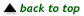Translations of mathematical formulas for web display were created by tex4ht. © Copyright 2003 Education Development Center, Inc. (EDC)